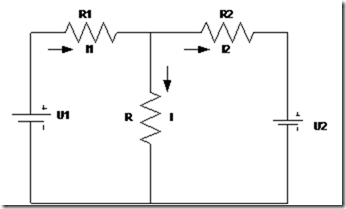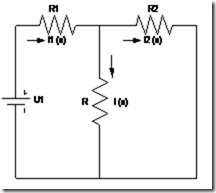" /> Theory of Superposition - TN Elektro
Home > English > Theory of Superposition

# Theory of Superposition

A linear network can consist of passive resistive element with two or more sources direct voltage. To analyze the circuit or network like this, it can be used the theory of superposition. In principle this theory utilizing a linear relationship between current and voltage. According to this theory, if there is a voltage source to work together in any linear network. Then any voltage source to work free of the influence of other voltage source.

Considered other voltage source does not exist, then the current in each penghamtar is composed of several components of the flow, where each component, each generated by each source voltage is contained in the circuit. Similarly, the voltage on each conductor is the algebraic sum of voltages generated by each voltage source independently.

Definition:
The current flowing in each branch of the circuit caused by a few pieces of voltage source contained in the circuit, is the algebraic sum of the currents in the branches, if the voltage source work independently. Each source voltage in the circuit considered to be working on their own, while the other voltage source can temporarily short circuited, then calculated the current flowing. The total current flowing in the branch is the algebraic sum of all the components of the current.

Example 1:
Sebua given a series of combinations as shown below. The circuit consists of three resistors, and two voltage sources. Determine the current (I) flowing in the resistor R.Solution:
Step 1: Suber voltage U2 short circuited, as follows:Step 2: The source voltage U1 short circuited as follows:In step one voltage U2 short circuited, then the current on each branch circuit is calculated. In step one voltage U1 short circuited, then the current on each branch circuit is calculated. Then in the can:
I1 = I1 (a) – I1 (b)
I2 = I2 (a) – I2 (b)
`I = I (a) – I (b)